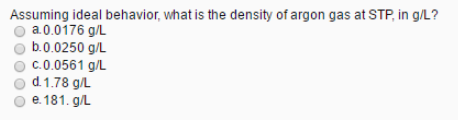# Problem: Assuming ideal behavior, what is the density of argon gas at STP, in g/L? a. 0.0176 g/L b. 0.0250 g/L c. 0.0561 g/L d. 1.78 g/L e. 181. g/L

###### FREE Expert Solution
97% (46 ratings)###### Problem Details

Assuming ideal behavior, what is the density of argon gas at STP, in g/L?

a. 0.0176 g/L

b. 0.0250 g/L

c. 0.0561 g/L

d. 1.78 g/L

e. 181. g/L